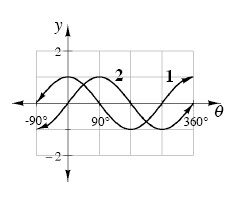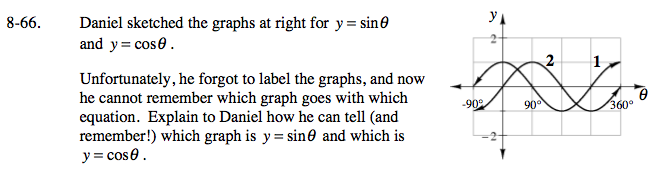Home > A2C > Chapter 8 > Lesson 8.1.4 > Problem8-66

8-66.

Daniel sketched the graphs below for y = sin θ and y = cos θ.
Unfortunately, he forgot to label the graphs, and now he cannot remember which graph goes with which equation. Explain to Daniel how he can tell (and remember!) which graph is y = sin θ and which is y = cos θ. Homework Help ✎Look at the y-intercepts. What is cos(0°)? sin(0°)?